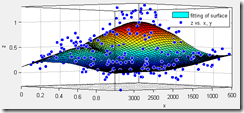# 多元回归 / 多元拟合matlab实现

MatLab内置了很多拟合的功能，比如分布、指数、多项式等拟合。对应的函数是fit。基本用法是：

cfun = fit(xdata, ydata, libname)

cfun = fit(x, y, ‘power1’)

ft = fittype( ‘a + b*sin(m*pi*x*y) + c*exp(-(w*y)^2)’, ‘indep’, {‘x’, ‘y’}, ‘depend’, ‘z’ );

opts = fitoptions( ft );

opts.Display = ‘Off’;

opts.Lower = [-Inf -Inf -Inf -Inf -Inf];

opts.StartPoint = [0.741864193163802 0.681571628918889 0.811658042300087 0.275716137656952 0.738979809065491];

opts.Upper = [Inf Inf Inf Inf Inf];

opts.Weights = zeros(1,0);

[fitresult, gof] = fit( [x, y], z, ft, opts );

b = regress(y, X)

beta = nlinfit(X,Y,modelfun,beta0)

beta = nlinfit(X,y,@hougen,beta0)

Matlab也提供了非线性多元拟合的图形化工具nlintool。